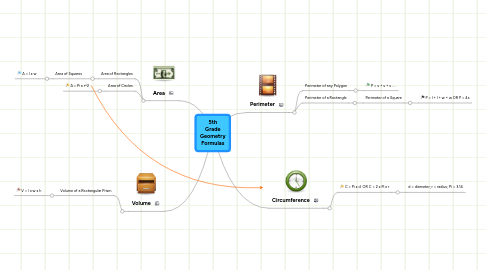# 5th Grade Geometry Formulas

Get Started. It's Free
or sign up with your email address5th Grade Geometry Formulas## 1. Perimeter

### 1.1. Perimeter of any Polygon

1.1.1. P = s + s + s . . .

### 1.2. Perimeter of a Rectangle

1.2.1. Perimeter of a Square

1.2.1.1. P = l + l + w + w OR P = 4s

## 2. Area

### 2.1. Area of Rectangles

2.1.1. Area of Squares

2.1.1.1. A = l x w

### 2.2. Area of Circles

2.2.1. A = Pi x r^2

## 3. Circumference

### 3.1. C = Pi x d OR C = 2 x Pi x r

3.1.1. d = diameter; r = radius; Pi = 3.14

## 4. Volume

### 4.1. Volume of a Rectangular Prism

4.1.1. V = l x w x h